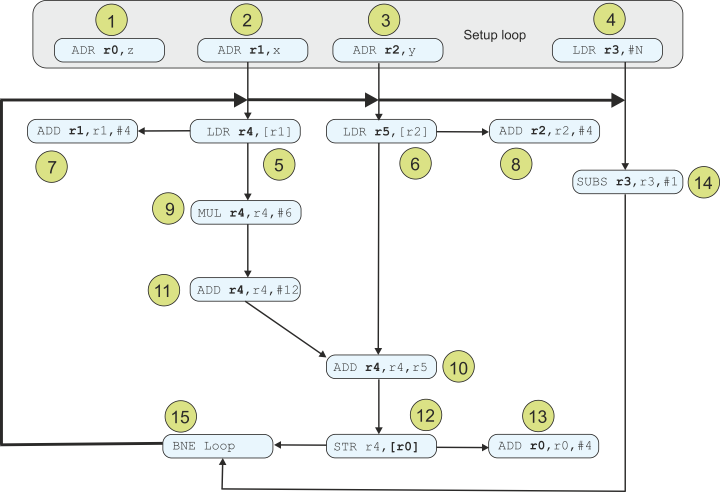﻿ Chap8 VLIW detail

A Discussion of Instruction Sequencing

The fundamental algorithm is:

4.       MOV   r3,#N     ;N is the loop count divided by 3

5. Loop  LDR   r4,[r1]   ;get x

6.       LDR   r5,[r2]   ;get y

7.       ADD   r1,r1,#4  ;increment x pointer

8.       ADD   r2,r2,#4  ;increment y pointer

9.       MUL   r4,r4,#6  ;6x

10.      ADD   r4,r4,r5  ;6x + y

11.      ADD   r4,r4,#12 ;6x + y + 12

12.      STR   r4,[r0]   ;store z

13.      ADD   r0,r0,#4  ;increment z pointer

14.      SUBS  r3,r3,#1  ;decrement loop counter

15.      BNE   Loop

This figure shows the sequencing of these instruction in terms of dependency. Note that an instruction on the same level connected by an arrow means that the instruction can be carried out at the same time or after. This applies to the pointer update instructions (e.g., 12 and 13) because a pointer can be used concurrently with its updating.

The thick line in this figure is the repeat loop from the branch to the start of a loop. This figure shows that some instructions such as 7, 5, 6, 8, and 14 can all be carried out in parallel. However, instructions 5, 9, 10, 11, and 12 must be carried out serially. Because we can perform (6x + y) + 12 or (6x + 12) + y, instructions 10 and 11 can be executed 10,11 or 11,10.

Load Store Branch                                             Data processing                                             Data processing

Stall no operation          ADR   r2,Y                 MOV   r3,#N

LDR   r4,[r1]               ADD   r1,r1,#4             Stall no operation

Stall load                  Stall no operation         Stall no operation

LDR   r5,[r2]               MUL   r4,r4,#6             ADD   r2,r2,#4

Stall load                  Stall multiply             Stall no operation

Stall no operation          ADD   r4,r4,r5             Stall no operation

Stall no operation          ADD   r4,r4,#12            Stall no operation

STR   r4,[r0]               ADD   r0,r0,#4             SUBS  r3,r3,#1

BNE   Loop                  Stall no operation         Stall no operation